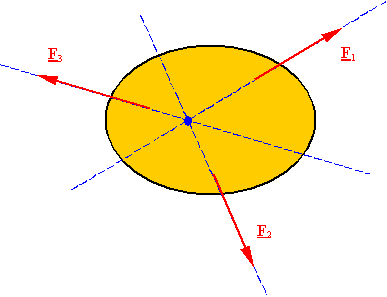Equilibrium of rigid bodies

Static equilibrium for a rigid body: A body (or any part of it) which is currently stationary will remain stationary if the resultant force and resultant moment are zero for all the forces and couples applied on it.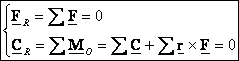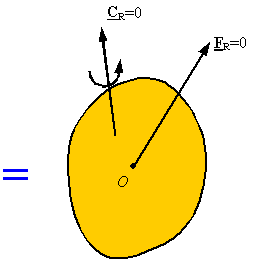Newton’s 3rd law: Each action has a reaction equal in magnitude and opposite in direction. This law provides the method used for one body (or part of a body) to interact with another body (or another part of the body).

Free-Body Diagram: A diagram of a body (or a part of it) which shows all the forces and couples applied on it, and which has all the forces and couples labeled for use in the solution of the problem is called a free-body diagram. Follow these steps to draw a free-body diagram.

1.      Select the body (or  part of a body) that you want to analyze, and draw it.

2.      Identify all the forces and couples that are applied onto the body and draw them on the body. Place each force and couple at the point that it is applied.

3.      Label all the forces and couples with unique labels for use during the solution process.

Composite bodies and internal forces:  Forces and couples which are a result of interaction between one part of an object and another part of it will not appear in the free-body diagram of the whole object. This is due to Newton’s 3rd law. The two bodies in the following example are welded at A. When the two parts are looked at as a single body, the internal forces and couples are added together, and as a result of Newton’s third law will cancel.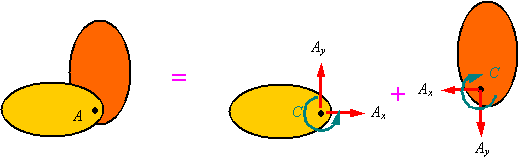Forces and couples on a free-body diagram: Each force or couple you put on a free-body diagram represents a model of how the body in the free-body diagram is effected by its surroundings. In selecting the forces and couples that are to be applied on the free-body diagram follow these steps:

1.      Identify all the forces which come from the interaction of one body with another. Many of the common supports and their effects are shown in Table 5-1 on page 184. Remember that for each way in which a support restricts the free motion of the body, a force or a moment must be applied to the body to impose the restriction on the motion.

2.      Apply the weight of the body to its center of gravity (if it is uniform, then apply it to the centroid).

3.      Remember that strings and cables can only pull on an object.

4.      Remember that internal loads cancel out and should not be put on the free-body diagram.

5.      Remember that if you have selected the direction of forces or couples of interaction on one body, then Newton’s 3rd law states that you must apply the forces or couples in the opposite direction on the other body.

Solving for unknowns: You can write one set of equilibrium equations for each free-body diagram you draw. Things to remember are:

1.      In 2-D problems the equilibrium equations result in three independent scalar equations (two components of force and one component of moment). Therefore, you can only solve for three scalar unknowns.

2.      Try to select the point you take moments around such that the line of action of at least one unknown force passes through that point. This will eliminate one unknown from your moment equation and will result in simpler equations to work with. This step is not essential, but will significantly simplify the algebra involved in solving your system of equations.

3.      You can sometimes take moments about two or three different points in a problem. Select each point so that you eliminate one or more unknowns from the resulting moment equation. Remember that the additional equations you generate in this way are not independent of the original equations, and, therefore, you will still have only three independent equations in 2-D problems per free-body diagram and you can only solve for three unknowns per free-body diagram.

4.      For a composite body, if you have drawn a free-body diagram and written the equilibrium equations for each of its subsections, you will gain no additional information if you draw the free-body diagram of the entire composite body and write its equilibrium equations.

5.      In 3-D problems the equilibrium equations result in six independent scalar equations (three components of force and three components of moment). Therefore, you can solve for up to six scalar unknowns per free-body diagram.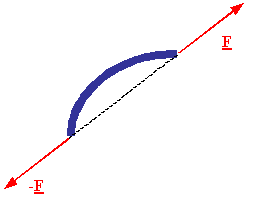A two-force member: A body which has forces  applied onto it at only two points, and no couples applied onto it at all, is called a two-force member. A two-force member can only be in equilibrium if the line of action of the resultant of the forces at each point passes through the other point, and each resultant force is equal in magnitude but opposite in direction to the resultant of the forces applied to the other point.

A three-force member: A body which has forces  applied onto it at only three points, and no couples applied onto it at all, is called a three-force member. A three-force member can only be in equilibrium if the lines of action for the resultants of the forces at each point intersect at a single point.